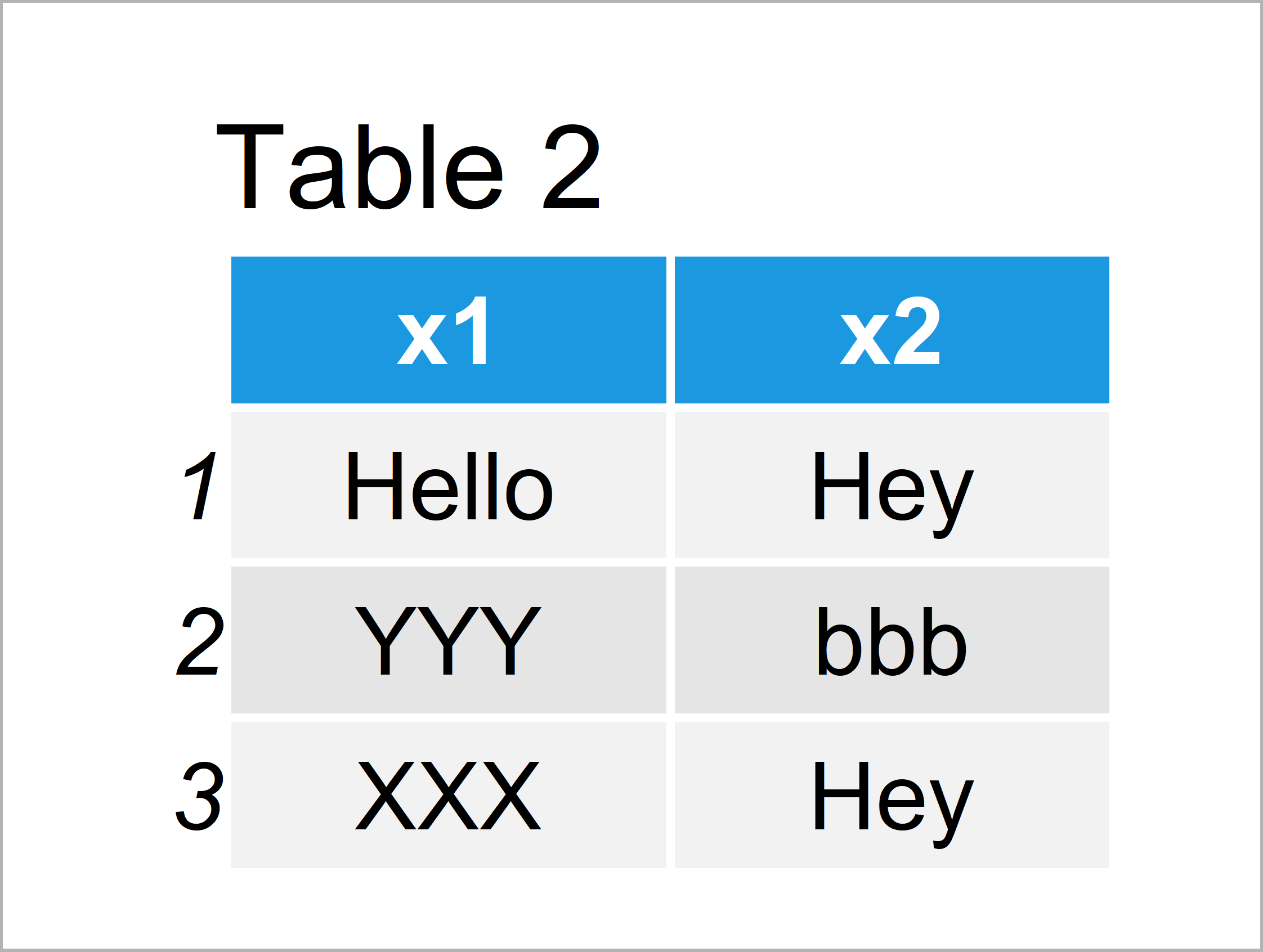# Replace Values in Factor Vector or Column in R (3 Examples)

In this article you’ll learn how to replace certain elements or entire levels of a factor in the R programming language.

The content looks as follows:

You’re here for the answer, so let’s get straight to the tutorial…

## Example 1: Replace Element in Factor Vector

In this example, I’ll show how to substitute specific elements of a vector with the factor class in R.

First, we have to create an example vector:

```x <- factor(c(LETTERS[1:5], "C"))                           # Create example factor vector
x                                                           # Print example factor vector
#  A B C D E C
# Levels: A B C D E```

Our example vector contains of six vector elements and five different factor levels.

Next, I’ll illustrate the problem with the replacement of contents in factors. Have a look at the following R code and the resulting warning message:

```x_warning <- x                                              # Duplicate example vector
x_warning <- "New"                                       # Try to replace factor value
# Warning message:
# In `[<-.factor`(`*tmp*`, 3, value = "New") :
#   invalid factor level, NA generated```

As you can see, the previous R code lead to the warning message invalid factor level, NA generated.

The reason for this is that we have tried to introduce the new factor level “New”. However, it is not possible to just insert new factor levels to an existing factor.

The following code shows that we have not inserted the new factor level “New”, but an NA value:

```x_warning                                                   # Print factor with NA
#  A    B    <NA> D    E    C
# Levels: A B C D E```

So how can we handle this problem? That’s what I’ll explain next!

The following R code converts our example vector to the character class first, replaces the value, and then converts the vector back to the factor class:

```x_new1 <- x                                                 # Duplicate example vector
x_new1 <- as.character(x_new1)                              # Convert factor vector to character
x_new1 <- "New"                                          # Replace specific value
x_new1 <- as.factor(x_new1)                                 # Convert character vector to factor
x_new1                                                      # Print updated vector
#  A   B   New D   E   C
# Levels: A B C D E New```

Have a look at the previous output of the RStudio console. As you can see, we have replaced the vector element at the third index position with the new factor level “New”.

## Example 2: Replace Level in Factor Vector

The following code illustrates how to exchange an entire factor level, i.e. all values that have this factor level.

For this, we can use the levels function as shown below:

```x_new2 <- x                                                 # Duplicate example vector
levels(x_new2)[levels(x_new2) == "C"] <- "New"              # Replace entire factor level
x_new2                                                      # Print updated vector
#  A   B   New D   E   New
# Levels: A B New D E```

The previous output shows that we have replaced all vector elements that had the factor level “C” with the factor level “New”.

## Example 3: Replace Factor Element & Level Columns of Data Frame

We can apply the same type of R code to data frame columns to exchange certain elements or entire factor column levels.

First, we have to create some example data:

```data <- data.frame(x1 = factor(c("XXX", "YYY", "XXX")),     # Create example data frame
x2 = factor(c("aaa", "bbb", "aaa")))
data                                                        # Print example data frame```In Table 1 it is shown that we have created a data frame with two columns.

Let’s duplicate these data to retain an original input data set:

`data_new <- data                                            # Duplicate example data frame`

Next, we can modify a particular value of the variable x1 with the same approach as in Example 1:

```data_new\$x1 <- as.character(data_new\$x1)                    # Convert data frame column to character
data_new\$x1 <- "Hello"                                   # Replace specific value
data_new\$x1 <- as.factor(data_new\$x1)                       # Convert data frame column to factor```

We can also apply the approach that we have used in Example 2 to change a whole factor level of a data frame column:

`levels(data_new\$x2)[levels(data_new\$x2) == "aaa"] <- "Hey"  # Replace entire factor level`

Let’s have a look at our new data frame:

`data_new                                                    # Print new data frame`As shown in Table 2, we have constructed a data table where we have changed a specific factor value in the column x1 and an entire factor level in the column x2.

## Video & Further Resources

Would you like to learn more about factors in R? Then I recommend having a look at the following video of my YouTube channel. In the video, I illustrate the topics of this tutorial.

Please accept YouTube cookies to play this video. By accepting you will be accessing content from YouTube, a service provided by an external third party.If you accept this notice, your choice will be saved and the page will refresh.

Furthermore, you could have a look at some of the other tutorials on my website. You can find a selection of tutorials below:

Summary: At this point you should know how to substitute factor elements and levels in the R programming language. If you have further questions, don’t hesitate to let me know in the comments section below.

Subscribe to the Statistics Globe Newsletter